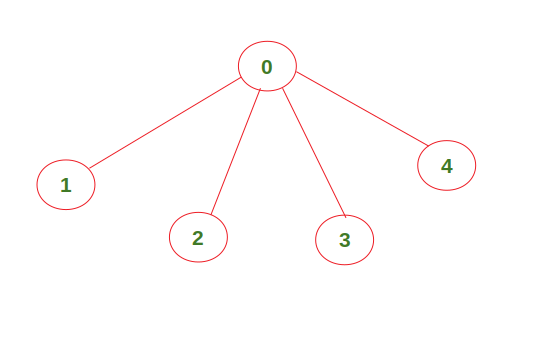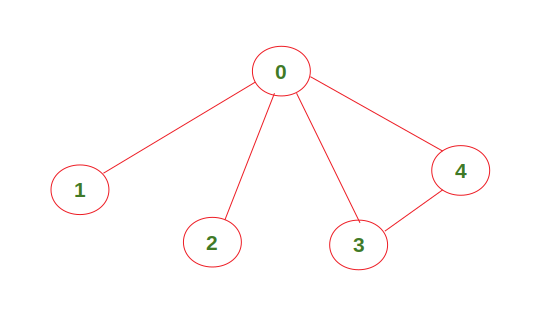# Find if an undirected graph contains an independent set of a given size

• Difficulty Level : Medium
• Last Updated : 07 Apr, 2022

Given an undirected graph, check if it contains an independent set of size k. Print ‘Yes’ if there exists an independent set of size k. Print ‘No’ otherwise.

Independent Set: An independent set in a graph is a set of vertices that are not directly connected to each other.

Example 1:

```Input : K = 4,
graph = [[1, 1, 0, 0, 0],
[1, 1, 1, 1, 1],
[0, 1, 1, 0, 0],
[0, 1, 0, 1, 0],
[0, 1, 0, 0, 1]]
Output : Yes ```The above graph contains an independent set of size 4 (vertices 1, 2, 3, 4 are not directly connected to each other). Hence, the output is ‘Yes’.

Example 2:

```Input : K = 4,
graph = [[1, 1, 0, 0, 0],
[1, 1, 1, 1, 1],
[0, 1, 1, 0, 0],
[0, 1, 0, 1, 1],
[0, 1, 0, 1, 1]]
Output : No```The above graph doesn’t contain an independent set of size 4. Hence, the output is ‘No’.

Approach:

• Initialize a variable sol with boolean False value.
• Find all the possible sets of vertices of size K from the given graph.
• If an independent set of size k is found, change the value of sol to True and return.
• Else continue checking for other possible sets.
• In the end, if sol is True, print ‘Yes’ else print ‘No’.

Below is the implementation of the above approach:

## C++14

 `// C++ code to check if a given graph` `// contains an independent set of size k` `#include ` `using` `namespace` `std;`   `// Function prototype` `bool` `check(``int``[], vector<``int``> &, ``int``);`   `// Function to construct a set of given size k` `bool` `func(``int` `graph[], vector<``int``> &arr, ` `          ``int` `k, ``int` `index, ``bool` `sol[])` `{` `    ``// Check if the selected set is independent or not.` `    ``// Change the value of sol to True and return` `    ``// if it is independent` `    ``if` `(k == 0)` `    ``{` `        ``if` `(check(graph, arr, arr.size()))` `        ``{` `            ``sol = ``true``;` `            ``return` `true``;` `        ``}` `    ``}` `    ``else` `    ``{` `        ``// Set of size k can be formed even if we don't` `        ``// include the vertex at current index.` `        ``if` `(index >= k)` `        ``{` `            ``vector<``int``> newvec(arr.begin(), arr.end());` `            ``newvec.push_back(index);` `            ``return` `(func(graph, newvec, k - 1, ` `                                ``index - 1, sol) or` `                    ``func(graph, arr, k, index - 1, sol));` `        ``}`   `        ``// Set of size k cannot be formed if we don't` `        ``// include the vertex at current index.` `        ``else` `        ``{` `            ``arr.push_back(index);` `            ``return` `func(graph, arr, k - 1, ` `                          ``index - 1, sol);` `        ``}` `    ``}` `}`   `// Function to check if the given set is` `// independent or not` `// arr --> set of size k (contains the` `// index of included vertex)` `bool` `check(``int` `graph[], vector<``int``> &arr, ``int` `n)` `{` `    ``// Check if each vertex is connected to any other` `    ``// vertex in the set or not` `    ``for` `(``int` `i = 0; i < n; i++)` `        ``for` `(``int` `j = i + 1; j < n; j++)` `            ``if` `(graph[arr[i]][arr[j]] == 1)` `                ``return` `false``;` `    ``return` `true``;` `}`   `// Driver Code` `int` `main()` `{` `    ``int` `graph[] = {{1, 1, 0, 0, 0},` `                      ``{1, 1, 1, 1, 1},` `                      ``{0, 1, 1, 0, 0},` `                      ``{0, 1, 0, 1, 0},` `                      ``{0, 1, 0, 0, 1}};` `    ``int` `k = 4;` `    ``vector<``int``> arr; ``// Empty set` `    ``bool` `sol[] = {``false``};` `    ``int` `n = ``sizeof``(graph) / ` `            ``sizeof``(graph);` `    ``func(graph, arr, k, n - 1, sol);`   `    ``if` `(sol)` `        ``cout << ``"Yes"` `<< endl;` `    ``else` `        ``cout << ``"No"` `<< endl;` `    ``return` `0;` `}`   `// This code is contributed by` `// sanjeev2552`

## Java

 `// Java code to check if a ` `// given graph contains an ` `// independent set of size k` `import` `java.util.*;` `class` `GFG{`   `static`  `Vector arr = ` `        ``new` `Vector<>();`   `// Function to cona set of ` `// given size k` `static` `boolean` `func(``int` `graph[][], ` `                    ``int` `k, ``int` `index, ` `                    ``boolean` `sol[])` `{` `  ``// Check if the selected set is ` `  ``// independent or not. Change the ` `  ``// value of sol to True and return` `  ``// if it is independent` `  ``if` `(k == ``0``)` `  ``{` `    ``if` `(check(graph, ` `              ``arr.size()))` `    ``{` `      ``sol[``0``] = ``true``;` `      ``return` `true``;` `    ``}` `  ``}` `  ``else` `  ``{` `    ``// Set of size k can be` `    ``// formed even if we don't ` `    ``// include the vertex at ` `    ``// current index.` `    ``if` `(index >= k)` `    ``{` `      ``Vector newvec = ` `             ``new` `Vector<>();` `      ``newvec.add(index);` `      ``return` `(func(graph, k - ``1``, ` `                   ``index - ``1``, sol) ||` `              ``func(graph, k, ` `                   ``index - ``1``, sol));` `    ``}`   `    ``// Set of size k cannot be ` `    ``// formed if we don't include ` `    ``// the vertex at current index.` `    ``else` `    ``{` `      ``arr.add(index);` `      ``return` `func(graph, k - ``1``, ` `                  ``index - ``1``, sol);` `    ``}` `  ``}` `  ``return` `true``;` `}`   `// Function to check if the ` `// given set is independent ` `// or not arr -. set of size ` `// k (contains the index of ` `// included vertex)` `static` `boolean` `check(``int` `graph[][], ` `                     ``int` `n)` `{` `  ``// Check if each vertex is ` `  ``// connected to any other` `  ``// vertex in the set or not` `  ``for` `(``int` `i = ``0``; i < n; i++)` `    ``for` `(``int` `j = i + ``1``; j < n; j++)` `      ``if` `(graph[arr.get(i)][arr.get(j)] == ``1``)` `        ``return` `false``;` `  ``return` `true``;` `}`   `// Driver Code` `public` `static` `void` `main(String[] args)` `{` `  ``int` `graph[][] = {{``1``, ``1``, ``0``, ``0``, ``0``},` `                   ``{``1``, ``1``, ``1``, ``1``, ``1``},` `                   ``{``0``, ``1``, ``1``, ``0``, ``0``},` `                   ``{``0``, ``1``, ``0``, ``1``, ``0``},` `                   ``{``0``, ``1``, ``0``, ``0``, ``1``}};` `  ``int` `k = ``4``;` `  ``boolean` `[]sol = ``new` `boolean``[``1``];` `  ``int` `n = graph.length;` `  ``func(graph, k, n - ``1``, sol);`   `  ``if` `(sol[``0``])` `    ``System.out.print(``"Yes"` `+ ``"\n"``);` `  ``else` `    ``System.out.print(``"No"` `+ ``"\n"``);` `}` `}`   `// This code is contributed by Amit Katiyar`

## Python3

 `# Python3 code to check if a given graph` `# contains an independent set of size k`   `# Function to construct a set of given size k` `def` `func(graph, arr, k, index, sol):` `    `  `    ``# Check if the selected set is independent or not. ` `    ``# Change the value of sol to True and return ` `    ``# if it is independent` `    ``if` `k ``=``=` `0``:` `        ``if` `check(graph, arr) ``=``=` `True``:` `            ``sol[``0``] ``=` `True` `            ``return` `        `  `    ``else``:` `        ``# Set of size k can be formed even if we don't ` `        ``# include the vertex at current index.` `        ``if` `index >``=` `k:` `            ``return` `(func(graph, arr[:] ``+` `[index], k``-``1``, index``-``1``, sol) ``or` `                    ``func(graph, arr[:], k, index``-``1``, sol))` `        `  `        ``# Set of size k cannot be formed if we don't` `        ``# include the vertex at current index.` `        ``else``:` `            ``return` `func(graph, arr[:] ``+` `[index], k``-``1``, index``-``1``, sol)`   `# Function to check if the given set is ` `# independent or not` `# arr --> set of size k (contains the` `# index of included vertex)` `def` `check(graph, arr):` `    `  `    ``# Check if each vertex is connected to any other` `    ``# vertex in the set or not` `    ``for` `i ``in` `range``(``len``(arr)):` `        ``for` `j ``in` `range``(i ``+` `1``, ``len``(arr)):` `            `  `            ``if` `graph[arr[i]][arr[j]] ``=``=` `1``:` `                ``return` `False` `                `  `    ``return` `True`   `# Driver Code    ` `graph ``=` `[[``1``, ``1``, ``0``, ``0``, ``0``],` `         ``[``1``, ``1``, ``1``, ``1``, ``1``],` `         ``[``0``, ``1``, ``1``, ``0``, ``0``],` `         ``[``0``, ``1``, ``0``, ``1``, ``0``],` `         ``[``0``, ``1``, ``0``, ``0``, ``1``]]` `        `  `k ``=` `4` `arr ``=` `[]     ``# Empty set ` `sol ``=` `[``False``]`   `func(graph, arr[:], k, ``len``(graph)``-``1``, sol)`   `if` `sol[``0``] ``=``=` `True``:` `    ``print``(``"Yes"``)` `else``:` `    ``print``(``"No"``)`

## C#

 `// C# code to check if a ` `// given graph contains an ` `// independent set of size k` `using` `System;` `using` `System.Collections.Generic;`   `class` `GFG{`   `static` `List<``int``> arr = ``new` `List<``int``>();`   `// Function to cona set of ` `// given size k` `static` `bool` `func(``int` `[,]graph, ` `                 ``int` `k, ``int` `index, ` `                 ``bool` `[]sol)` `{` `  `  `  ``// Check if the selected set is ` `  ``// independent or not. Change the ` `  ``// value of sol to True and return` `  ``// if it is independent` `  ``if` `(k == 0)` `  ``{` `    ``if` `(check(graph, ` `              ``arr.Count))` `    ``{` `      ``sol = ``true``;` `      ``return` `true``;` `    ``}` `  ``}` `  ``else` `  ``{` `    `  `    ``// Set of size k can be` `    ``// formed even if we don't ` `    ``// include the vertex at ` `    ``// current index.` `    ``if` `(index >= k)` `    ``{` `      ``List<``int``> newvec = ``new` `List<``int``>();` `      ``newvec.Add(index);` `      `  `      ``return` `(func(graph, k - 1, ` `                       ``index - 1, sol) ||` `              ``func(graph, k, ` `                   ``index - 1, sol));` `    ``}` `    `  `    ``// Set of size k cannot be ` `    ``// formed if we don't include ` `    ``// the vertex at current index.` `    ``else` `    ``{` `      ``arr.Add(index);` `      `  `      ``return` `func(graph, k - 1, ` `                     ``index - 1, sol);` `    ``}` `  ``}` `  ``return` `true``;` `}`   `// Function to check if the ` `// given set is independent ` `// or not arr -. set of size ` `// k (contains the index of ` `// included vertex)` `static` `bool` `check(``int` `[,]graph, ``int` `n)` `{` `  `  `  ``// Check if each vertex is ` `  ``// connected to any other` `  ``// vertex in the set or not` `  ``for``(``int` `i = 0; i < n; i++)` `    ``for``(``int` `j = i + 1; j < n; j++)` `      ``if` `(graph[arr[i],arr[j]] == 1)` `        ``return` `false``;` `  `  `  ``return` `true``;` `}`   `// Driver Code` `public` `static` `void` `Main(String[] args)` `{` `  ``int` `[,]graph = { { 1, 1, 0, 0, 0 },` `                   ``{ 1, 1, 1, 1, 1 },` `                   ``{ 0, 1, 1, 0, 0 },` `                   ``{ 0, 1, 0, 1, 0 },` `                   ``{ 0, 1, 0, 0, 1 } };` `  ``int` `k = 4;` `  ``bool` `[]sol = ``new` `bool``;` `  ``int` `n = graph.GetLength(0);` `  `  `  ``func(graph, k, n - 1, sol);`   `  ``if` `(sol)` `    ``Console.Write(``"Yes"` `+ ``"\n"``);` `  ``else` `    ``Console.Write(``"No"` `+ ``"\n"``);` `}` `}`   `// This code is contributed by Amit Katiyar`

## Javascript

 ``

Output:

`Yes`

Time Complexity:where V is the number of vertices in the graph and k is the given size of set.
Auxiliary Space: O(V)

My Personal Notes arrow_drop_up
Recommended Articles
Page :